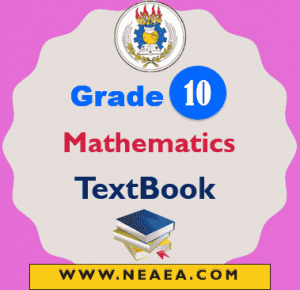# Ethiopian Grade 10 Mathematics Textbook For Students [PDF]

The Ethiopian Grade 10 Mathematics Textbook contains seven-unit and each unit has its subunits. At the end of each unit should should complete the Key Terms, Summary, and Review Exercises. Besides at end of this textbook there two tables i.e. Table of Trigonometric Functions and Table of Common Logarithms.## Ethiopian Grade 10 Mathematics Student Textbook

Mathematics learning in grade 10 has to significantly contribute to the fulfillment of the main objectives of learning mathematics. In this respect, developing high-level abilities and competencies in calculating is one of the main tasks of learning Grade 10 mathematics.

Students should be in a position to tackle problems in an effective way, including estimations, rough calculations, and checking the exactness of the result. The use of polynomial, exponential, logarithmic and trigonometric functions for describing phenomena in nature and society and solving respective real-life problems should be realized and appreciated by students

Unit 1: Polynomial Functions

• 1.1 Introduction to polynomial functions
• 1.2 Theorems on polynomials
• 1.3 Zeros of a polynomial function
• 1.4 Graphs of polynomial functions.
Key Terms.
Summary
Review Exercises on Unit 1

Unit 2: Exponential and Logarithmic Functions

• 2.1 Exponents and logarithms
• 2.2 The exponential functions and their graphs
• 2.3 The logarithmic functions and their graphs
• 2.4 Equations involving exponents and logarithms
• 2.5 Applications of exponential and logarithmic functions
Key Terms
Summary
Review Exercises on Unit 2

Unit: 3 Solving Inequalities

• 3.1 Inequalities involving absolute value
• 3.2 Systems of liner inequalities in two variables
Key Terms
Summary
Review Exercises on Unit 3

Unit 4: Coordinate Geometry

• 4.1 Distance between two points
• 4.2 Division of a line segment
• 4.3 Equation of a line
• 4.4 Parallel and perpendicular lines
Key Terms
Summary
Review Exercises on Unit 4

Unit 5: Trigonometric Functions

• 5.1 Basic trigonometric functions
• 5.2 The reciprocal functions of the basic trigonometric functions
• 5.3 Simple trigonometric identities
• 5.4 Real life application problems
Key Terms
Summary
Review Exercises on Unit 5

Unit 6: Plane Geometry

• 6.1 Theorems on triangles
• 6.3 More on circles
• 6.4 Regular polygons
Key Terms
Summary
Review Exercises on Unit 6

Unit 7: Measurement

• 7.1 Revision on surface areas and volumes of prisms and cylinders
• 7.2 Pyramids, cones and spheres
• 7.3 Frustums of pyramids and cones
• 7.4 Surface areas and volumes of composed solids
Key Terms
Summary
Review Exercises on Unit 7

Table of Trigonometric Functions
Table of Common Logarithms